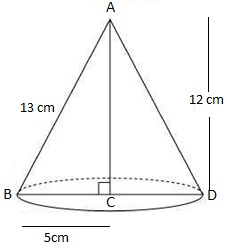Question

# A right triangle ABC with sides $5 cm, 12 cm$ and $13 cm$ is revolved about the side $12 cm$. Find the volume of the solid so obtained.13em 12cm

Open in App
Solution

## Given that a right triangle ABC with sides $5cm,12cm$ and $13cm$ is revolved about the side $12cm$So, after revolution a cone will be formed, such thatRadius of cone $\left(r\right)=5cm$Height of cone $\left(h\right)=12cm$Now, Volume of cone $=\frac{1}{3}×{\mathrm{\pi r}}^{2}\mathrm{h}$ $=\frac{1}{3}×\mathrm{\pi }×5×5×12\phantom{\rule{0ex}{0ex}}=\frac{12×\mathrm{\pi }×5×5}{3}\phantom{\rule{0ex}{0ex}}\mathrm{V}=100\mathrm{\pi }{\mathrm{cm}}^{3}$Therefore, Volume of the cone$=100\mathrm{\pi }$ $c{m}^{3}$Suggest Corrections19Similar questions